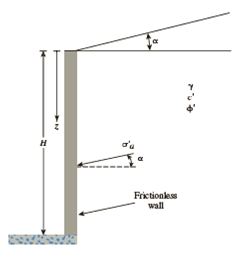Chapter 13, Problem 13.21PPrinciples of Geotechnical Enginee...

9th Edition
Braja M. Das + 1 other
ISBN: 9781305970939

Solutions

Chapter
SectionPrinciples of Geotechnical Enginee...

9th Edition
Braja M. Das + 1 other
ISBN: 9781305970939
Textbook Problem

Figure 13.13 shows a frictionless wall with a sloping granular backfill. Given: H = 7 m, α = 12°, ϕ ′ = 28°, and γ = 18.6 kN/m3.a. Determine the magnitude of active pressure, σ ′ a , at the bottom of the wall.b. Determine Rankine active force, Pa, per unit length of the wall and its location and direction.Figure 13.13 Frictionless vertical retaining wall with sloping backfill

(a)

To determine

Find the magnitude of active pressure σa at the bottom of the wall.

Explanation

Given information:

The height (H) of the retaining wall is 7.0 m.

The soil friction angle ϕ is 28°.

The angle α is 12°.

The unit weight γ of the backfill is 18.6kN/m3.

Calculation:

Calculate magnitude of active pressure σa at the bottom of the wall using the relation.

σa=γzKa

Here, Ka is the active earth pressure coefficient.

Refer Table 13.2 “Values of K” in the text book.

Take the value of active earth pressure coefficient for the given soil friction angle 28° and for the angle 12° as 0

(b)

To determine

Find the Rankine active force Pa per unit length of the wall and its location and direction.

Still sussing out bartleby?

Check out a sample textbook solution.

See a sample solution

The Solution to Your Study Problems

Bartleby provides explanations to thousands of textbook problems written by our experts, many with advanced degrees!

Get Started

What is Hadoop, and what are its basic components?

Database Systems: Design, Implementation, & Management

What are two reasons for center drilling on the lathe?

Precision Machining Technology (MindTap Course List)

Define an actor, and provide three examples.

Systems Analysis and Design (Shelly Cashman Series) (MindTap Course List)

Knowing that the resultant of the two forces is vertical, determine the angle

International Edition---engineering Mechanics: Statics, 4th Edition

What are methods to protect computers, mobile devices, and networks from attacks? (218)

Enhanced Discovering Computers 2017 (Shelly Cashman Series) (MindTap Course List)